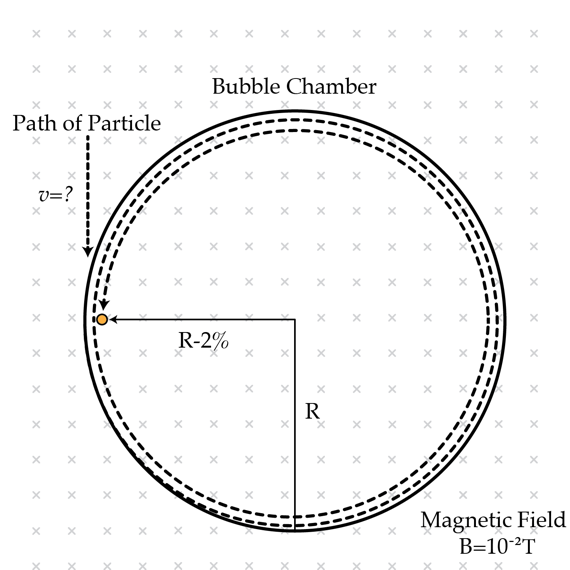# A particle spiraling in a magnetic field

A particle with specific charge $\alpha:= \frac{q}{m}=10^{8} \textrm{ C/kg}$ enters a bubble chamber where its path can be observed. A magnetic field of induction $B=10^{-2} \textrm{T}$ is perpendicular to the particle's velocity. The particle experiences a viscous drag force which is approximately proportional to its velocity, that is $\vec{F}_{drag}=- k \vec{v}.$ As a consequence of this force, the particle spirals inward. It is observed that after two full rotations the radius of curvature decreases by $2 \%$. Then the magnetic field is switched off and the particle travels $L=30 \textrm{cm}$ before coming to a stop. What was the particle's velocity in m/s right before entering the chamber?×

Problem Loading...

Note Loading...

Set Loading...